# Chapter 6 Pipes

## 6.1 Introduction

R code contain a lot of parentheses in case of a sequence of multiple operations. When you are dealing with complex code, it results in nested function calls which are hard to read and maintain. The magrittr package by Stefan Milton Bache provides pipes enabling us to write R code that is readable.

Pipes allow us to clearly express a sequence of multiple operations by:

• structuring operations from left to right
• avoiding
• nested function calls
• intermediate steps
• overwriting of original data
• minimizing creation of local variables

## 6.2 Pipes

If you are using tidyverse, magrittr will be automatically loaded. We will look at 3 different types of pipes:

• `%>%` : pipe a value forward into an expression or function call
• `%<>%`: result assigned to left hand side object instead of returning it
• `%\$%` : expose names within left hand side objects to right hand side expressions

We will use the following R packages:

``````library(magrittr)
library(dplyr)
library(stringr)
library(purrr)``````

## 6.3 Data

``````ecom <-
col_types = cols_only(
referrer = col_factor(levels = c("bing", "direct", "social", "yahoo", "google")),
n_pages = col_double(), duration = col_double(), purchase = col_logical()
)
)

ecom``````
``````## # A tibble: 1,000 x 4
##    referrer n_pages duration purchase
##    <fct>      <dbl>    <dbl> <lgl>
##  1 google         1      693 FALSE
##  2 yahoo          1      459 FALSE
##  3 direct         1      996 FALSE
##  4 bing          18      468 TRUE
##  5 yahoo          1      955 FALSE
##  6 yahoo          5      135 FALSE
##  7 yahoo          1       75 FALSE
##  8 direct         1      908 FALSE
##  9 bing          19      209 FALSE
## 10 google         1      208 FALSE
## # ... with 990 more rows``````

We will create a smaller data set from the above data to be used in some examples:

``````ecom_mini <- sample_n(ecom, size = 10)
ecom_mini``````
``````## # A tibble: 10 x 4
##    referrer n_pages duration purchase
##    <fct>      <dbl>    <dbl> <lgl>
##  1 direct         1      141 FALSE
##  2 social        14      406 FALSE
##  3 direct         1      896 FALSE
##  4 bing           9      261 FALSE
##  5 direct        11      154 FALSE
##  6 direct         1      296 FALSE
##  7 yahoo          1      594 FALSE
##  8 social        12      300 TRUE
##  9 bing          10      260 TRUE
## 10 bing          19      209 FALSE``````

### 6.3.1 Data Dictionary

• referrer: referrer website/search engine
• n_pages: number of pages visited
• duration: time spent on the website (in seconds)
• purchase: whether visitor purchased

## 6.5 Square Root

Time to try a slightly more challenging example. We want the square root of `n_pages` column from the data set.

``y <- sqrt(ecom_mini\$n_pages)``

Let us break down the above computation into small steps:

• select/expose the `n_pages` column from `ecom` data
• compute the square root
• assign the first few observations to `y`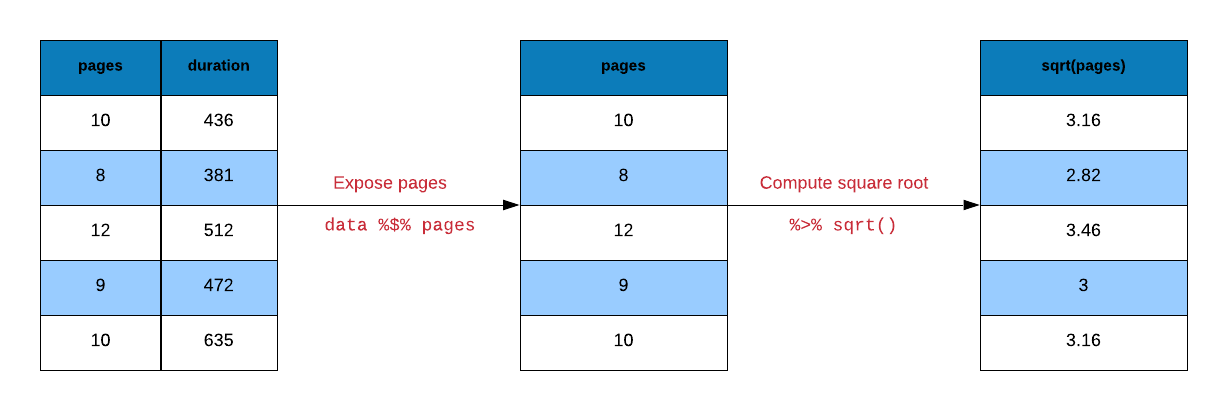Let us reproduce `y` using pipes.

``````# select n_pages variable and assign it to y
y <-
ecom_mini %\$%
n_pages

# compute square root of y and assign it to y
y %<>% sqrt``````

Another way to compute the square root of y is shown below.

``````y <-
ecom_mini %\$%
n_pages %>%
sqrt()``````

## 6.6 Visualization

Let us look at a data visualization example. We will create a bar plot to visualize the frequency of different referrer types that drove purchasers to the website. Let us look at the steps involved in creating the bar plot:

• extract rows where purchase is TRUE
• select/expose `referrer` column
• tabulate referrer data using `table()`
• use the tabulated data to create bar plot using `barplot()`
``barplot(table(subset(ecom, purchase)\$referrer))``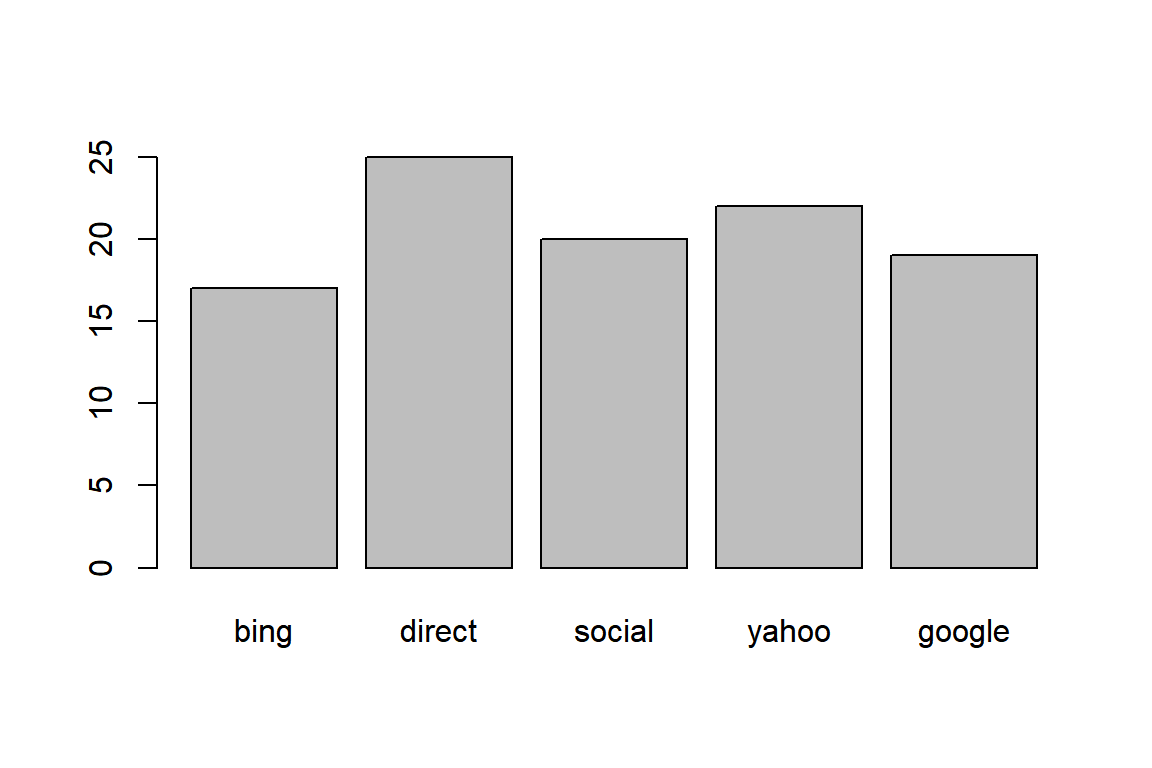### 6.6.1 Using pipe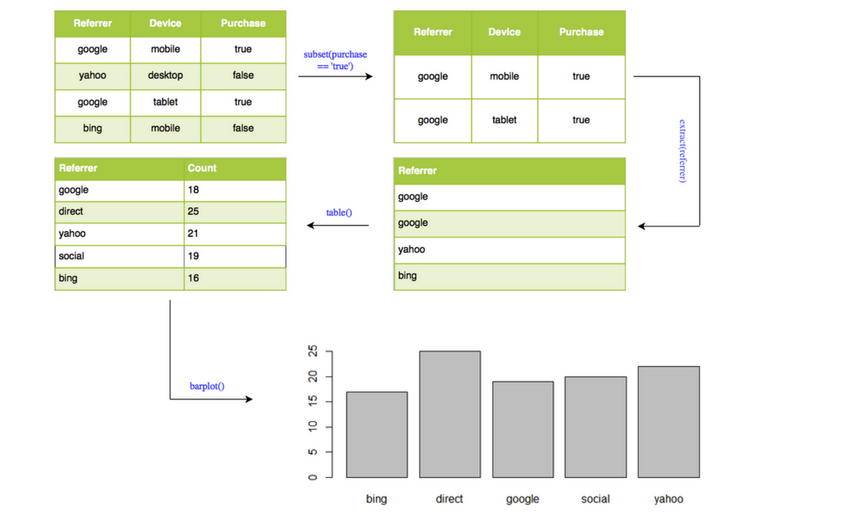``````ecom %>%
subset(purchase) %>%
extract('referrer') %>%
table() %>%
barplot()``````## 6.7 Correlation

Correlation is a statistical measure that indicates the extent to which two or more variables fluctuate together. In R, correlation is computed using `cor()`. Let us look at the correlation between the number of pages browsed and time spent on the site for visitors who purchased some product. Below are the steps for computing correlation:

• extract rows where purchase is TRUE
• select/expose `n_pages` and `duration` columns
• use `cor()` to compute the correlation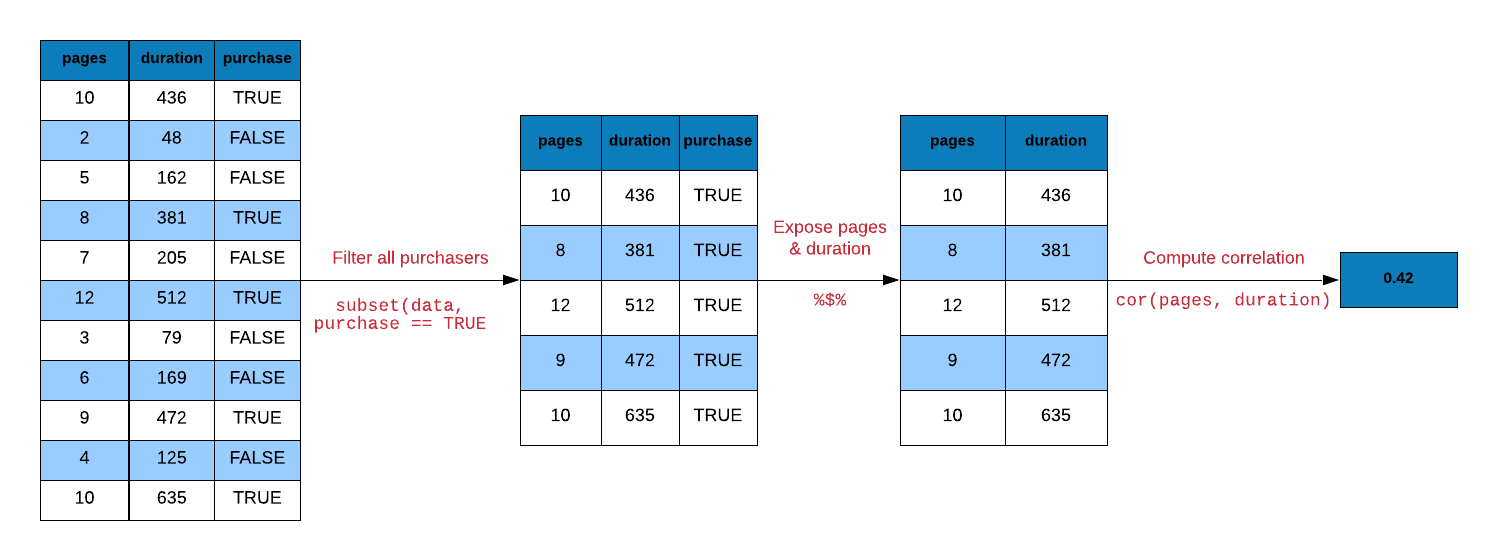``````# without pipe
ecom1 <- subset(ecom, purchase)
cor(ecom1\$n_pages, ecom1\$duration)``````
``##  0.4290905``
``````# with pipe
ecom %>%
subset(purchase) %\$%
cor(n_pages, duration)``````
``##  0.4290905``
``````# with pipe
ecom %>%
filter(purchase) %\$%
cor(n_pages, duration)``````
``##  0.4290905``

## 6.8 Regression

Let us look at a regression example. We regress time spent on the site on number of pages visited. Below are the steps involved in running the regression:

• use `duration` and `n_pages` columns from ecom data
• pass the above data to `lm()`
• pass the output from `lm()` to `summary()`
``summary(lm(duration ~ n_pages, data = ecom))``
``````##
## Call:
## lm(formula = duration ~ n_pages, data = ecom)
##
## Residuals:
##     Min      1Q  Median      3Q     Max
## -386.45 -213.03  -38.93  179.31  602.55
##
## Coefficients:
##             Estimate Std. Error t value Pr(>|t|)
## (Intercept)  404.803     11.323  35.750  < 2e-16 ***
## n_pages       -8.355      1.296  -6.449 1.76e-10 ***
## ---
## Signif. codes:  0 '***' 0.001 '**' 0.01 '*' 0.05 '.' 0.1 ' ' 1
##
## Residual standard error: 263.3 on 998 degrees of freedom
## Multiple R-squared:   0.04,  Adjusted R-squared:  0.03904
## F-statistic: 41.58 on 1 and 998 DF,  p-value: 1.756e-10``````

### 6.8.1 Using pipe

``````ecom %\$%
lm(duration ~ n_pages) %>%
summary()``````
``````##
## Call:
## lm(formula = duration ~ n_pages)
##
## Residuals:
##     Min      1Q  Median      3Q     Max
## -386.45 -213.03  -38.93  179.31  602.55
##
## Coefficients:
##             Estimate Std. Error t value Pr(>|t|)
## (Intercept)  404.803     11.323  35.750  < 2e-16 ***
## n_pages       -8.355      1.296  -6.449 1.76e-10 ***
## ---
## Signif. codes:  0 '***' 0.001 '**' 0.01 '*' 0.05 '.' 0.1 ' ' 1
##
## Residual standard error: 263.3 on 998 degrees of freedom
## Multiple R-squared:   0.04,  Adjusted R-squared:  0.03904
## F-statistic: 41.58 on 1 and 998 DF,  p-value: 1.756e-10``````

## 6.9 String Manipulation

We want to extract the first name (jovial) from the below email id and convert it to upper case. Below are the steps to achieve this:

• split the email id using the pattern `@` using `str_split()`
• extract the first element from the resulting list using `extract2()`
• extract the first element from the character vector using `extract()`
• extract the first six characters using `str_sub()`
• convert to upper case using `str_to_upper()`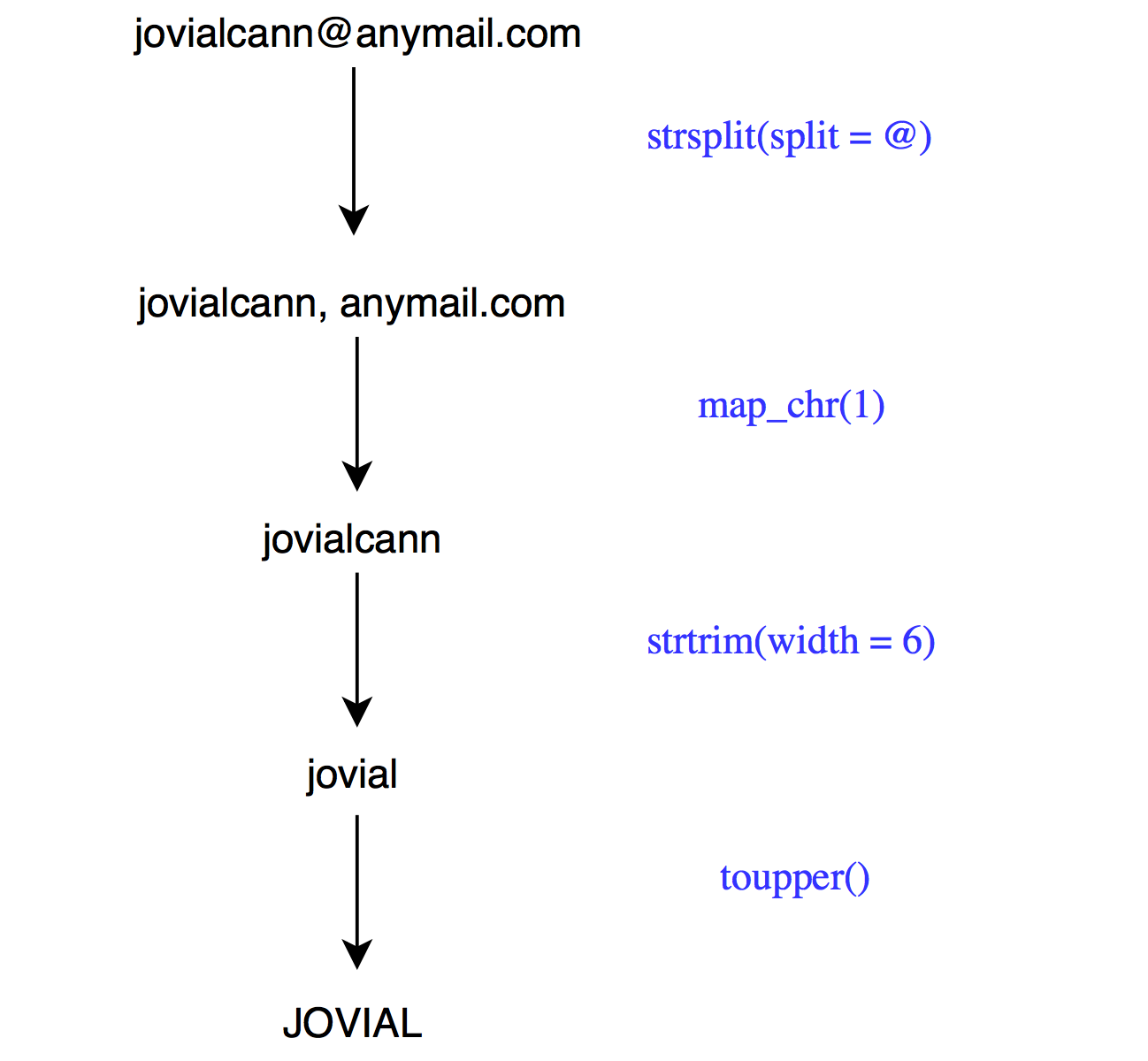``````email <- 'jovialcann@anymail.com'

# without pipe
str_to_upper(str_sub(str_split(email, '@')[], start = 1, end = 6))``````
``##  "JOVIAL"``
``````# with pipe
email %>%
str_split(pattern = '@') %>%
extract2(1) %>%
extract(1) %>%
str_sub(start = 1, end = 6) %>%
str_to_upper()``````
``##  "JOVIAL"``

Another method that uses `map_chr()` from the purrr package.

``````email %>%
str_split(pattern = '@') %>%
map_chr(1) %>%
str_sub(start = 1, end = 6) %>%
str_to_upper()``````
``##  "JOVIAL"``

## 6.10 Data Extraction

Let us turn our attention towards data extraction. magrittr provides alternatives to `\$`, `[` and `[[`.

• `extract()`
• `extract2()`
• `use_series()`

### 6.10.1 Extract Column

To extract a specific column using the column name, we mention the name of the column in single/double quotes within `[` or `[[`. In case of `\$`, we do not use quotes.

``````# base
ecom_mini['n_pages']``````
``````## # A tibble: 10 x 1
##    n_pages
##      <dbl>
##  1       1
##  2      14
##  3       1
##  4       9
##  5      11
##  6       1
##  7       1
##  8      12
##  9      10
## 10      19``````
``````# magrittr
extract(ecom_mini, 'n_pages') ``````
``````## # A tibble: 10 x 1
##    n_pages
##      <dbl>
##  1       1
##  2      14
##  3       1
##  4       9
##  5      11
##  6       1
##  7       1
##  8      12
##  9      10
## 10      19``````

We can extract columns using their index position. Keep in mind that index position starts from 1 in R. In the below example, we show how to extract `n_pages` column but instead of using the column name, we use the column position.

``````# base
ecom_mini``````
``````## # A tibble: 10 x 1
##    n_pages
##      <dbl>
##  1       1
##  2      14
##  3       1
##  4       9
##  5      11
##  6       1
##  7       1
##  8      12
##  9      10
## 10      19``````
``````# magrittr
extract(ecom_mini, 2) ``````
``````## # A tibble: 10 x 1
##    n_pages
##      <dbl>
##  1       1
##  2      14
##  3       1
##  4       9
##  5      11
##  6       1
##  7       1
##  8      12
##  9      10
## 10      19``````

One important differentiator between `[` and `[[` is that `[[` will return a atomic vector and not a `data.frame`. `\$` will also return a atomic vector. In magrittr, we can use `use_series()` in place of `\$`.

``````# base
ecom_mini\$n_pages``````
``##    1 14  1  9 11  1  1 12 10 19``
``````# magrittr
use_series(ecom_mini, 'n_pages') ``````
``##    1 14  1  9 11  1  1 12 10 19``

### 6.10.2 Extract List Element

Let us convert `ecom_mini` into a list using as.list() as shown below:

``ecom_list <- as.list(ecom_mini)``

To extract elements of a list, we can use `extract2()`. It is an alternative for `[[`.

``````# base
ecom_list[['n_pages']]``````
``##    1 14  1  9 11  1  1 12 10 19``
``````# magrittr
extract2(ecom_list, 'n_pages')``````
``##    1 14  1  9 11  1  1 12 10 19``
``````# base
ecom_list[]``````
``````##   direct social direct bing   direct direct yahoo  social bing   bing
## Levels: bing direct social yahoo google``````
``````# magrittr
extract2(ecom_list, 1)``````
``````##   direct social direct bing   direct direct yahoo  social bing   bing
## Levels: bing direct social yahoo google``````

We can extract the elements of a list using `use_series()` as well.

``````# base
ecom_list\$n_pages``````
``##    1 14  1  9 11  1  1 12 10 19``
``````# magrittr
use_series(ecom_list, n_pages)``````
``##    1 14  1  9 11  1  1 12 10 19``

## 6.11 Arithmetic Operations

magrittr offer alternatives for arithemtic operations as well. We will look at a few examples below.

• `add()`
• `subtract()`
• `multiply_by()`
• `multiply_by_matrix()`
• `divide_by()`
• `divide_by_int()`
• `mod()`
• `raise_to_power()`

``1:10 + 1``
``##    2  3  4  5  6  7  8  9 10 11``
``add(1:10, 1)``
``##    2  3  4  5  6  7  8  9 10 11``
```+`(1:10, 1)``
``##    2  3  4  5  6  7  8  9 10 11``

### 6.11.2 Multiplication

``1:10 * 3``
``##    3  6  9 12 15 18 21 24 27 30``
``multiply_by(1:10, 3)``
``##    3  6  9 12 15 18 21 24 27 30``
```*`(1:10, 3)``
``##    3  6  9 12 15 18 21 24 27 30``

### 6.11.3 Division

``1:10 / 2``
``##   0.5 1.0 1.5 2.0 2.5 3.0 3.5 4.0 4.5 5.0``
``divide_by(1:10, 2)``
``##   0.5 1.0 1.5 2.0 2.5 3.0 3.5 4.0 4.5 5.0``
```/`(1:10, 2)``
``##   0.5 1.0 1.5 2.0 2.5 3.0 3.5 4.0 4.5 5.0``

### 6.11.4 Power

``1:10 ^ 2``
``````##      1   2   3   4   5   6   7   8   9  10  11  12  13  14  15  16  17  18
##    19  20  21  22  23  24  25  26  27  28  29  30  31  32  33  34  35  36
##    37  38  39  40  41  42  43  44  45  46  47  48  49  50  51  52  53  54
##    55  56  57  58  59  60  61  62  63  64  65  66  67  68  69  70  71  72
##    73  74  75  76  77  78  79  80  81  82  83  84  85  86  87  88  89  90
##    91  92  93  94  95  96  97  98  99 100``````
``raise_to_power(1:10, 2)``
``##     1   4   9  16  25  36  49  64  81 100``
```^`(1:10, 2)``
``##     1   4   9  16  25  36  49  64  81 100``

## 6.12 Logical Operators

There are alternatives for logical operators as well. We will look at a few examples below.

• `and()`
• `or()`
• `equals()`
• `not()`
• `is_greater_than()`
• `is_weakly_greater_than()`
• `is_less_than()`
• `is_weakly_less_than()`

### 6.12.1 Greater Than

``1:10 > 5``
``##   FALSE FALSE FALSE FALSE FALSE  TRUE  TRUE  TRUE  TRUE  TRUE``
``is_greater_than(1:10, 5)``
``##   FALSE FALSE FALSE FALSE FALSE  TRUE  TRUE  TRUE  TRUE  TRUE``
```>`(1:10, 5)``
``##   FALSE FALSE FALSE FALSE FALSE  TRUE  TRUE  TRUE  TRUE  TRUE``

### 6.12.2 Weakly Greater Than

``1:10 >= 5``
``##   FALSE FALSE FALSE FALSE  TRUE  TRUE  TRUE  TRUE  TRUE  TRUE``
``is_weakly_greater_than(1:10, 5)``
``##   FALSE FALSE FALSE FALSE  TRUE  TRUE  TRUE  TRUE  TRUE  TRUE``
```>=`(1:10, 5)``
``##   FALSE FALSE FALSE FALSE  TRUE  TRUE  TRUE  TRUE  TRUE  TRUE``